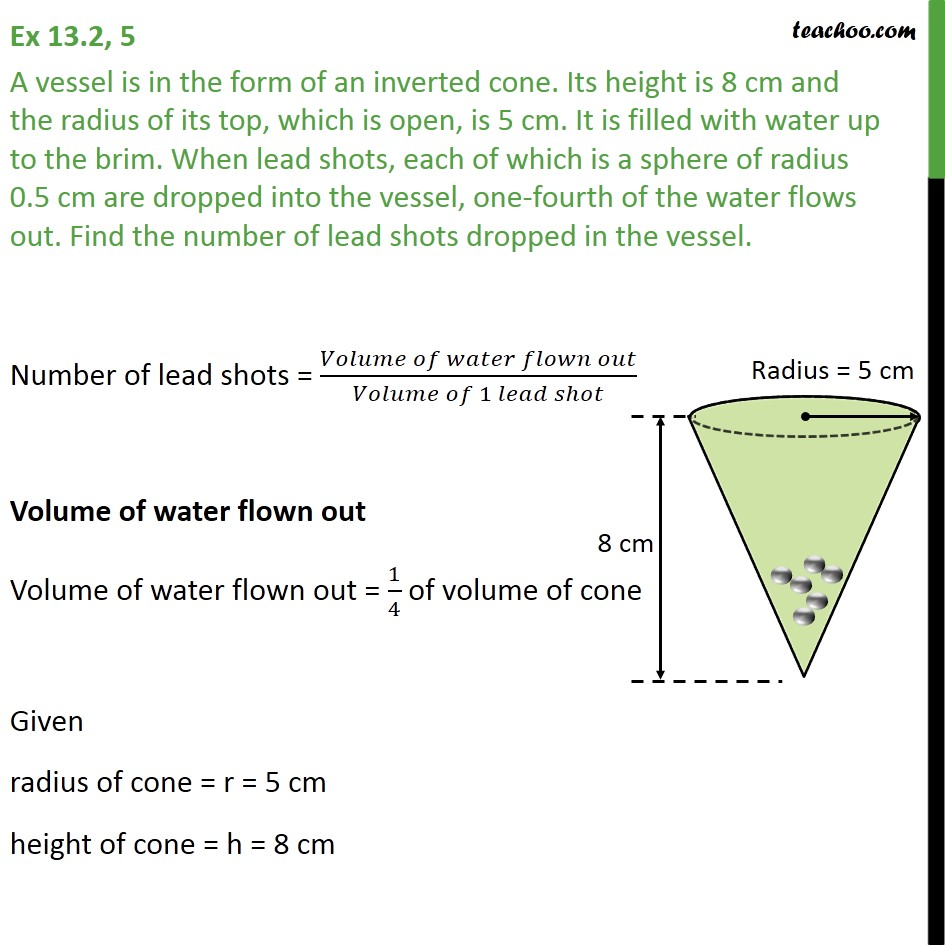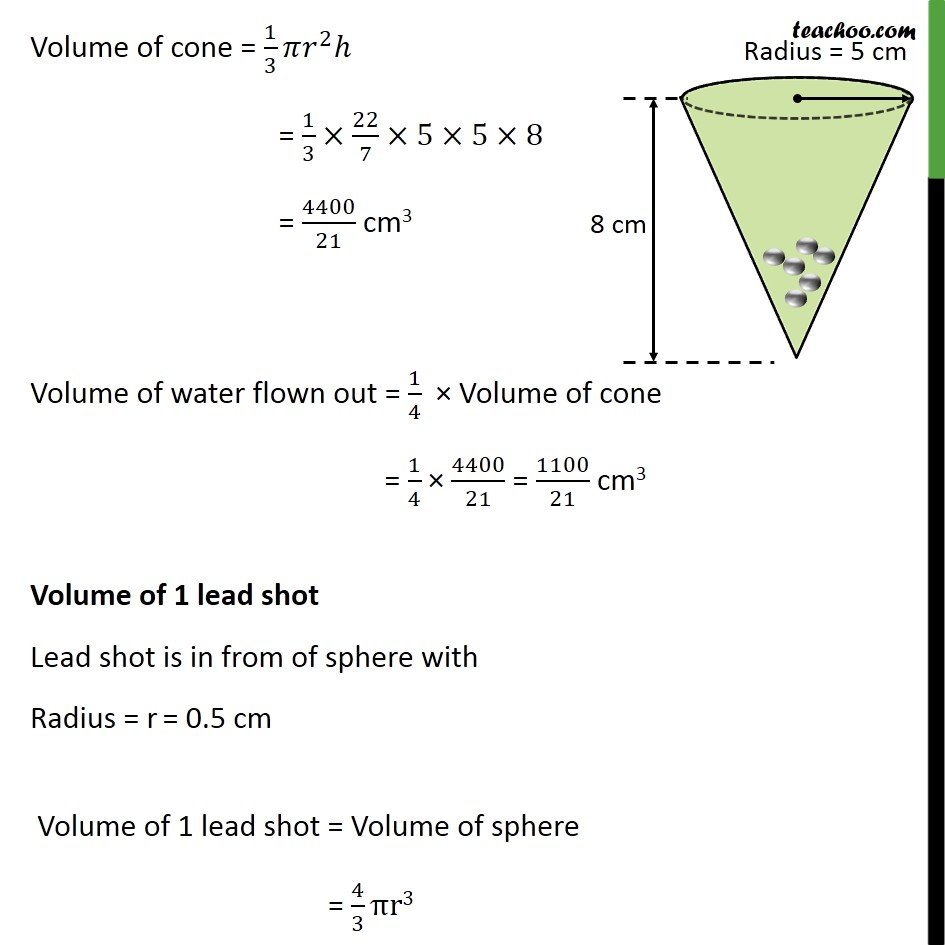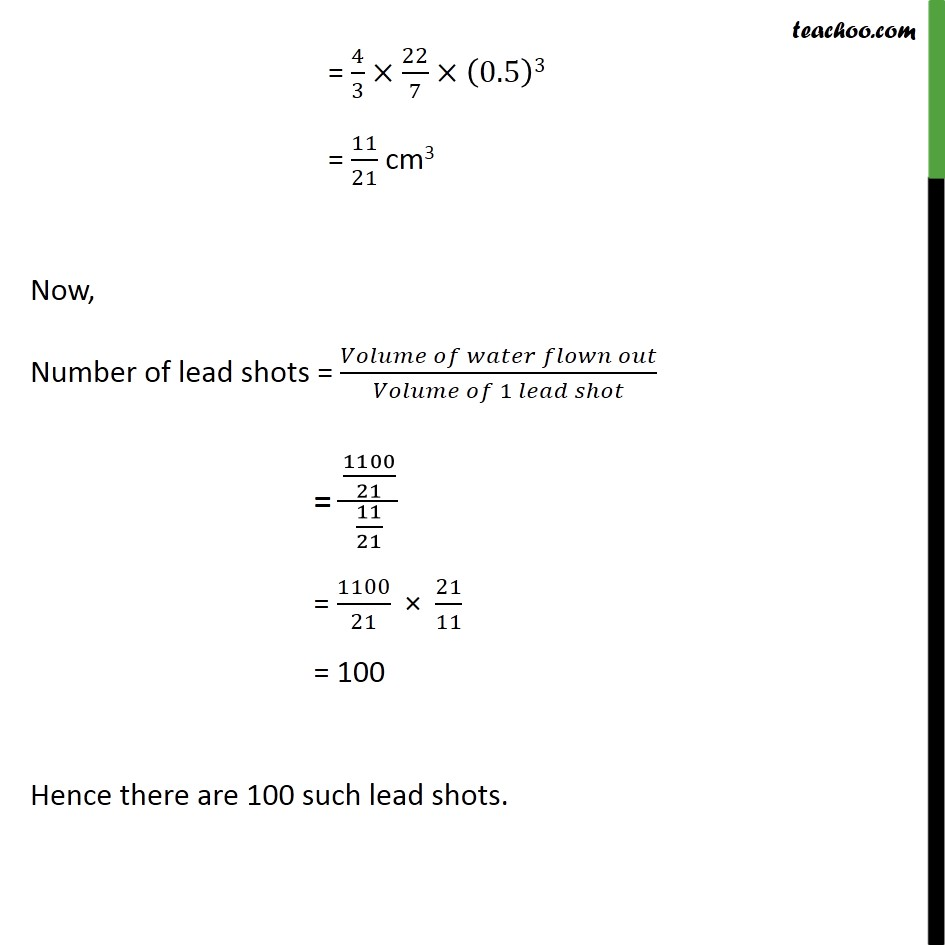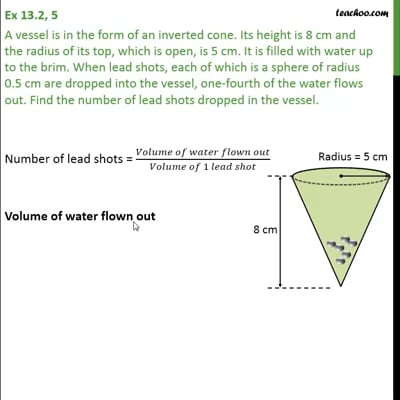Ex 13.2

Chapter 13 Class 10 Surface Areas and Volumes
Serial order wiseThis video is only available for Teachoo black users

Solve all your doubts with Teachoo Black (new monthly pack available now!)

### Transcript

Ex 13.2, 5 A vessel is in the form of an inverted cone. Its height is 8 cm and the radius of its top, which is open, is 5 cm. It is filled with water up to the brim. When lead shots, each of which is a sphere of radius 0.5 cm are dropped into the vessel, one-fourth of the water flows out. Find the number of lead shots dropped in the vessel. Number of lead shots = (𝑉𝑜𝑙𝑢𝑚𝑒 𝑜𝑓 𝑤𝑎𝑡𝑒𝑟 𝑓𝑙𝑜𝑤𝑛 𝑜𝑢𝑡)/(𝑉𝑜𝑙𝑢𝑚𝑒 𝑜𝑓 1 𝑙𝑒𝑎𝑑 𝑠ℎ𝑜𝑡) Volume of water flown out Volume of water flown out = 1/4 of volume of cone Given radius of cone = r = 5 cm height of cone = h = 8 cm Volume of cone = 1/3 𝜋𝑟^2 ℎ = 1/3×22/7×5×5×8 = 4400/21 cm3 Volume of water flown out = 1/4 × Volume of cone = 1/4 × 4400/21 = 1100/21 cm3 Volume of 1 lead shot Lead shot is in from of sphere with Radius = r = 0.5 cm Volume of 1 lead shot = Volume of sphere = 4/3 πr3 = 4/3×22/7×(0.5)3 = 11/21 cm3 Now, Number of lead shots = (𝑉𝑜𝑙𝑢𝑚𝑒 𝑜𝑓 𝑤𝑎𝑡𝑒𝑟 𝑓𝑙𝑜𝑤𝑛 𝑜𝑢𝑡)/(𝑉𝑜𝑙𝑢𝑚𝑒 𝑜𝑓 1 𝑙𝑒𝑎𝑑 𝑠ℎ𝑜𝑡) = (1100/21)/(11/21) = 1100/21 × 21/11 = 100 Hence there are 100 such lead shots.